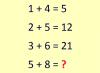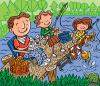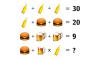BRAIN TEASERSBrain Teasers User Profile

 Calculate 5+8 If 1+4=5, 2+5=12 and 3+6=21 then 5+8=?There are 6 words hidden in ... There are 6 words hidden in the picture, can you find 1 of 6?MATH PUZZLE: Can you replace... MATH PUZZLE: Can you replace the question mark with a number?